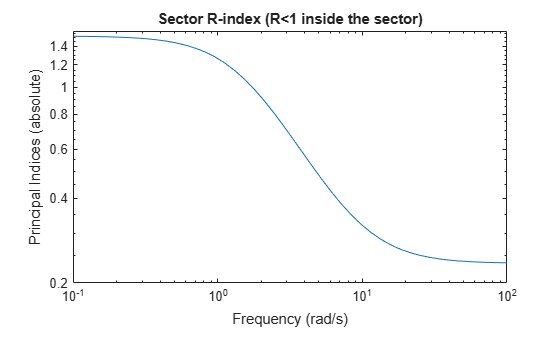getSectorCrossover

Crossover frequencies for sector bound

Description

example

wc = getSectorCrossover(H,Q) returns the frequencies at which the following matrix M(ω) is singular:

$M\left(\omega \right)=H{\left(j\omega \right)}^{H}Q\text{\hspace{0.17em}}H\left(j\omega \right).$

When a frequency-domain sector plot exists, these frequencies are the frequencies at which the relative sector index (R-index) for H and Q equals 1. See About Sector Bounds and Sector Indices for details.

Examples

collapse all

Find the crossover frequencies for the dynamic system $G\left(s\right)=\left(s+2\right)/\left(s+1\right)$ and the sector defined by:

$S=\left\{\left(y,u\right):a{u}^{2}

for various values of a and b.

In U/Y space, this sector is the shaded region of the following diagram (for a, b > 0).The Q matrix for this sector is given by:

$Q=\left[\begin{array}{cc}1& -\left(a+b\right)/2\\ -\left(a+b\right)/2& ab\end{array}\right];\phantom{\rule{1em}{0ex}}a=0.1,\phantom{\rule{0.2777777777777778em}{0ex}}b=10.$

getSectorCrossover finds the frequencies at which $H\left(s{\right)}^{H}QH\left(s\right)$ is singular, for $H\left(s\right)=\left[G\left(s\right);I\right]$. For instance, find these frequencies for the sector defined by Q with a = 0.1 and b = 10.

G = tf([1 2],[1 1]);
H = [G;1];

a = 0.1;
b = 10;
Q = [1 -(a+b)/2 ; -(a+b)/2 a*b];

w = getSectorCrossover(H,Q)
w =

0x1 empty double column vector

The empty result means that there are no such frequencies.

Now find the frequencies at which ${H}^{H}QH$ is singular for a narrower sector, with a = 0.5 and b = 1.5.

a2 = 0.5;
b2 = 1.5;
Q2 = [1 -(a2+b2)/2 ; -(a2+b2)/2 a2*b2];

w2 = getSectorCrossover(H,Q2)
w2 = 1.7321

Here the resulting frequency is where the R-index for H and Q2 is equal to 1, as shown in the sector plot.

sectorplot(H,Q2)Thus, when a sector plot exists for a system H and sector Q, getSectorCrossover finds the frequencies at which the R-index is 1.

Input Arguments

collapse all

Model to analyze against sector bounds, specified as a dynamic system model such as a tf, ss, or genss model. H can be continuous or discrete. If H is a generalized model with tunable or uncertain blocks, getSectorCrossover analyzes the current, nominal value of H.

To get the frequencies at which the I/O trajectories (u,y) of a linear system G lie in a particular sector, use H = [G;I], where I = eyes(nu), and nu is the number of inputs of G.

Sector geometry, specified as:

• A matrix, for constant sector geometry. Q is a symmetric square matrix that is ny on a side, where ny is the number of outputs of H.

• An LTI model, for frequency-dependent sector geometry. Q satisfies Q(s)’ = Q(–s). In other words, Q(s) evaluates to a Hermitian matrix at each frequency.

The matrix Q must be indefinite to describe a well-defined conic sector. An indefinite matrix has both positive and negative eigenvalues.

Output Arguments

collapse all

Sector crossover frequencies, returned as a vector. The frequencies are expressed in rad/TimeUnit, relative to the TimeUnit property of H. If the trajectories of H never cross the boundary, wc = [].